## Monday, August 17, 2015

### Astro-Stats : Bayes' Theorem (for Astrophysics), cont.

Now, it is time for us to apply what I explained in the previous post to Python code.  As always, you can follow along on your computer if you have Python installed.  You can copy my code (from here) and paste it into your own file, or you can download my file using the "wget" command in the Terminal. (And don't forget to get the necessary text file mentioned in the previous post.)

I will lead you into this exercise a little differently than I typically do.  I will first show you an unsatisfactory fitting.  This will allow you to see how the process towards finding a satisfactory fit goes sometimes.  After exhibiting the unsatisfactory result, we will learn from the previous results and then run my code again to find the better results.

>>>
>>>execfile('companion_detect.py')
>>>p_posterior(metal_0, metal_1, alphas=np.linspace(0,1,100), betas=np.linspace(0,1,120), plot=True)
>>>

As stated in the previous post, many priors (for each parameter) must be inserted to perform the Grid Search methodology.  When running the code, we have just input 100 different values for the $$\alpha$$ parameter that lie between 0 and 1.  120 numbers between 0 and 1 were chosen as the priors for $$\beta$$.  The contour plot shows the Likelihood for each combination of $$\alpha$$ and $$\beta$$.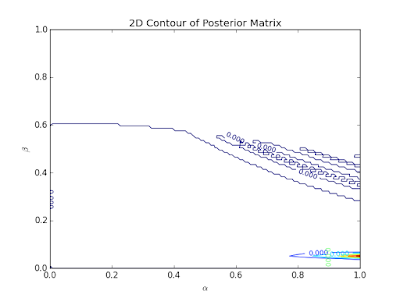Figure 1: Contour plot of Likelihoods for the two parameters.  Colors blue to red represent least to largest Likelihoods respectively.

I know what you're thinking... "This is an ugly plot.  Did something go wrong?"  Well, no.  Everything is fine with my code.  This ugliness insinuates that the priors assigned were far from correct.  Notice how the reddish area is barely visible?  This means that values near 1.0 for $$\alpha$$ yield higher Likelihoods than the blue or colorless regions.  The location of the red area also implies that values near 0.1 seem promising for the $$\beta$$ parameter.Figure 2: Terrible looking cumulative distribution function (CDF) plot.  This CDF was suppose to derive from a normal probability distribution function (PDF).  This plot suggests that the PDF was not of a normal distribution.  This is unfortunate primarily because the parameter and its uncertainties were chosen by assuming that the PDF was Gausssian.

If you want to understand the intricate details about how the plot of Figure 2 came about, read my Grad Level Intro... post and the Grad Level Intro... cont. post.  Those posts will also explain why the PDF and CDF are important.  For now, I'll just say that the purpose of Figure 2 is to provide the best fitting parameter.  There are two reasons why I am not satisfied with the result given by Figure 2.  The first reason for my disdain is the hideosity of the plots (and yes, that is a real word).  The second is the non-Gaussian PDF.  Figure 1 and 2 plots suggest that this fitting did not go well and that the unseen PDF is not of a normal distribution although it should be.Figure 3: Atrocious attempt at finding the best fitting value for $$\beta$$.
The disgusting plots of Figures 2 and 3 only confirm the terrible fitting job that was insinuated first by Figure 1.

Well, all is not lost!  As stated previously, the contour plot gave us a clue as to how we can ameliorate the fitting.  Now, let's run the code with different priors.

>>>
>>>execfile('companion_detect.py')
>>>p_posterior(metal_0, metal_1, alphas=np.linspace(0,0.1,100), betas=np.linspace(1,3,120), plot=True)

Now, our $$\alpha$$ priors range from 0 to 0.1 and our $$\beta$$ priors span from 1 to 3.  Let's check out the results!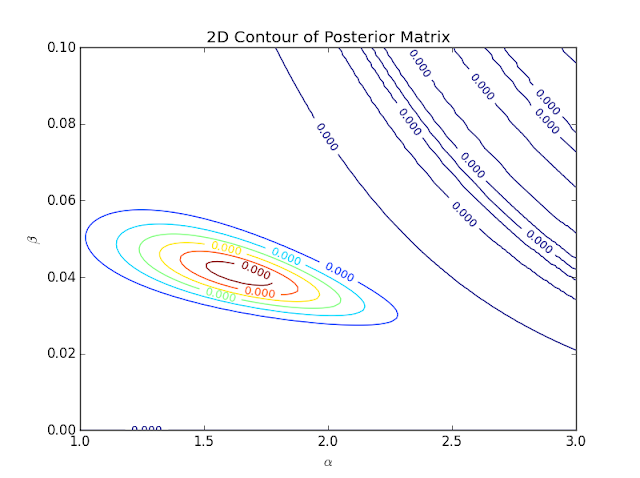Figure 4: Contour plot for the grid search methodology employed.  The Likelihoods calculated are so small that every number shown seems to be the same because only the first 4 digits are shown.  However, I know that the colors represent the different Likelihoods from blue (least) to red (greatest).
That contour plot looks much more aesthetic than the previous one.  However, the number situation can be very confusing at first glance.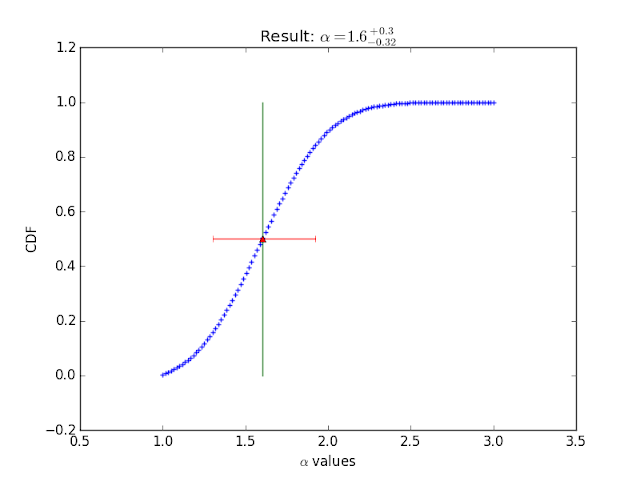Figure 5: Plot of the CDF for the $$\alpha$$ parameter.  The value yielding the best fit was 1.6 for this parameter.Figure 6: Plot of CDF for $$\beta$$ parameter.

There are your results!  Although, I do assume that the results would be more accurate if I were to constrain the range of priors more.

I wanted to show the bad fitting first as a way to convey the detective-like nature of astrophysics researchers.  Researchers, no matter how smart, almost never get the accurate or most satisfactory results on the first try.  This is why one must put on the detective hat and sniff for clues as to what might have gone wrong during the analysis.  I looked for clues in that first contour plot once I noticed how "fishy" it looked.  Figure 1 led me to choosing better priors for my second attempt at the Grid Search.  Moments like that reaffirm my love for plots.  Experiences like that have persuaded me to create many plots as I write complex code.  The graphics can really give a person the ability to see subtle issues that may be visually conspicuous in one's code or methodology that may not be discerned by merely glancing at a bunch of numbers on a computer screen.

## Monday, August 3, 2015

### Astro-Stats & Python : Bayes' Theorem (for Astrophysics)

Back to the Astro-Stats!

In my Bayes' Theorem (for a Coin) post, I introduced Bayes' Theorem for probability along with an example with a Python script.  This time, I will use the same concept for astrophysical data.  Let me first set the stage for this new example and then I'll get to the code so that you can follow along your computer.

My data comprises of the metallicity of stars and the confirmation/denial of a planet detection around a star.  You can see the text file containing this data here.  If you want to follow along, you must copy this information and paste it into your own text file; or, you can download this file using the "wget" command (on that same link) in the Terminal.Figure 1: Snapshot of part of table in the ".txt" file.  The first column is the real name of the star.  The second column is the metallicity of the star relative to the Sun.  This metallicity is the ratio of iron abundance to hydrogen abundance [Fe/H].  Because the Sun is used as the reference, Fe/H for the Sun equals 0.
As with my last analysis with Bayes' Theorem, I must input a prior for each parameter sought, find the Likelihood and then evaluate the new parameters.  In terms of mathematics, the differences between this exercise and the Coin example are conspicuously seen within my Likelihood and Bayes' Probability equations.

The Likelihood is equal to the probability (for the parameters' being measured) of all of the stars with detected planets multiplied by the probability (for the same parameters) of all of the stars without a planet detection.  Because each detection or absence thereof are events that occur independently of each other, the probabilities must be multiplied together to obtain the total probability for the stars with detected planets, as well as those without planets.  This can be described as
$\mathcal{L}=\prod^{k} P(\alpha,\beta) \cdot \prod^{N-k}[ 1-P(\alpha, \beta) ]$
where $$\mathcal{L}$$ is the Likelihood, k is the number of stars with planets, N is the number of stars total, P is the probability of stars with planets, and $$\alpha$$ and $$\beta$$ are parameters.  The rightmost $$\prod$$ term is the total probability of stars without detected planets and the $$\prod$$ term to the left of that is the total probability of stars with detected planets.

Essentially, the Likelihood is being found by multiplying two probabilities.  This corresponds to the generic definition of Likelihood, which can be written as $$\mathcal{L} = \prod^{N}_{i}P_{i}$$  where N is the total number of probabilities.  Likelihood can be defined as the product of all the probabilities.  This was discussed briefly in my Exercise in Likelihood and $$\chi^2$$, continued... post.

For this specific example, the given probability equation is defined as
$P (\alpha, \beta) = \alpha 10^{\beta M}$
where M is the metallicity of one of the observed stars.  Be aware that many statistical applications assume the probability of all outcomes to be equal to each other.  This equation signifies that the probability of each star for having a planet companion is distinct and depends primarily on the metallicity of that star along with the parameters.

Unlike the Coin example, I must now suggest many priors for my parameters $$\alpha$$ and $$\beta$$.  Previously, I provided only 1 prior probability and then let my function use the newfound posterior probability as the subsequent iteration's prior probability.  My methodology is different now because I will use the Grid Search and contour plot strategy to determine which of my priors yielded the highest Likelihoods and thus the highest posterior probabilities.  This method was used in my Grad Level to Intro to Astro-Stats for Undergraduates post and its sequel.  Similar to the equation stated for my Coin example, the posterior Likelihood is described as the following proportion.
$\mathcal{L}_{posterior} \propto \alpha \cdot \beta \cdot \mathcal{L}_{prior}$
Each $$\mathcal{L}_{posterior}$$ will be tracked inside of a matrix, or grid, of numbers that will later be used to create a contour plot.

Now that the math and concepts are (hopefully) clear to you, we can get to the real-life application of all of this knowledge.  My next post will illustrate the results of utilizing this statistical method in my Python script.

to be continued...

## Monday, July 20, 2015

### Someone you should know: Lauren Woolsey, cont.

Lauren Woolsey received her Bachelor's Degree at the University of Maryland, College Park.  While there, she proactively found opportunities that would satisfy her passion for astronomy.  One of these opportunities included an internship at the Goddard Space Flight Center (GSFC).  Conveniently, the GSFC is not far away from the university.  Lauren earned an internship there both her sophomore and senior year of college.  During these undergraduate years she was also occupied with her job as a Teaching Assistant during her junior year.  After that year, she landed an internship under the NSF-funded Solar REU program at the Harvard-Smithsonian Center for Astrophysics (CfA).

These accomplishments are but a few of many reasons why she was a strong applicant when applying for graduate schools during her senior year.  Consequently, she was accepted into the Astronomy Department of Harvard's Graduate School of Arts and Sciences.  Since then, she has been working with Steve Cranmer on modeling the influence of different magnetic field geometries on the solar wind.

When I spoke to Lauren about her professional background, I was intrigued by the diversity of her undergraduate research experiences.  At Goddard, she studied planetary surfaces and specifically analyzed topographical maps of Mars and the Moon to see how the rate of crater formation and history of impacts have affected certain locations.  At her undergraduate internship at the CfA, her project involved comparing and contrasting a solar wind model to observations recorded by the SOHO Ultraviolet Coronagraph Spectrometer.  At the end of her senior year, she finished her Honors thesis project in which she sought to explain the tilt of Uranus via a non-linear resonance in the different orbital and inclination frequencies of the solar system.

Now that she is a Ph.D. candidate, her research experiences are only getting more interesting.  For most of her graduate career as Steve Cranmer’s advisee, she has been modeling how magnetic fields affect solar wind.  One parameter that has been of interest to her is the magnetic field ratio between the photosphere and a region called the source surface. The source surface surrounds the Sun at a distance of 2.5 solar radii from the solar core.

Lauren is one of many astronomers who focus on the theory behind a phenomenon more than current data gathered via observations.  As a theorist, she has been working with models that attempt to imitate solar wind speed that has been observed in reality.  The field ratio has proven to be useful for modeling this solar wind speed and thus it’s also been beneficial towards finding a better correlation between the Sun’s magnetic field and solar wind properties.  Furthermore, finding a better correlation might even lead scientists to better comprehending one of the most famously unsolved problems in the astronomy community: why is the outermost atmospheric layer of the Sun hotter than most regions closer to the Sun’s extremely hot core?

What makes this enigma even more flabbergasting is that scientists know specifically where this phenomenon is taking place and still have yet to figure out this problem.  The region where the temperature of the Sun drastically increases is called the transition region.  The transition region dwells immediately above the chromosphere and occupies a thin layer of the Sun’s corona.  The solution to this problem seems to be occurring in this transition region; however, scientists do not currently know why it is happening and what mechanism, starting in the transition region or below, is causing the temperature to be so high in the corona.

Lauren started her search for the relation between the magnetic field and solar wind properties by working with one-dimensional models. The model represented the magnetic field strength as a function of height.  The footpoint of the model acted as the photosphere while the source region was the source surface.Figure 1: Plot of relationship between magnetic field strength and altitude.  The information comes from Lauren's one-dimensional modeling from photosphere to source surface.  The rightmost dashed line aligns with 1.50 on the x-axis, representing the source region (2.50 $$R_{\odot}$$ away from Sun’s core).  For a more detailed explanation of this plot, see Lauren's paper.
After working with that model she took it a step further and considered additional information from a higher-dimensional code with time dependence.  With this model, astronomers are given a more clear understanding of how significantly the magnetic field ratio affects certain properties of the solar wind.  This newfound understanding is primarily due to how well the model replicates events on the Sun that have been observed in reality.Figure 2: 3-dimensional model of magnetic field lines between footpoint (bottom of imaginary cylinder) and source region (top of cylinder).  According to the model, this is the configuration of the magnetic field lines at a specific moment in time. (van Ballegooijen et al. 2011)
Because modeling inevitably entails the comparison of the model to data measured in reality, Lauren’s most recent work involves data taken of network jets on the Sun.  Network jets are bright, short-lived, linear features in the chromosphere.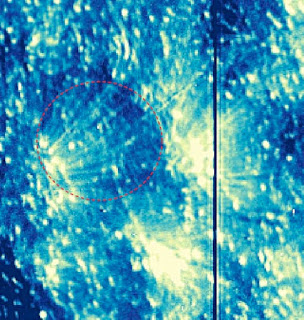Figure 3: Observation of solar network jets present within the red dashed circle. (Picture Credit: NASA/IRIS)
Lauren is currently conducting research on these solar network jets and hopes that her newfound knowledge will ameliorate her previous work with modeling or simply prove how well the models work. In turn, she will be able to improve the current understanding of the physics behind the correlation between magnetic field strength and solar wind properties.  While working on this she has managed to publish a paper this past March.  She has also finished another paper recently which will soon be published.  It is obvious that she knows and enjoys the essence of writing and communicating her research to the scientific community.  She is a testament to the fact that writing is an essential part of a researcher’s life.  That form of communication is vital to the productivity and knowledge of the astronomy community.  I hope to see her work continue to benefit the astronomy community as well as lead to breakthroughs in science that would ameliorate the well-being of all of mankind.

### Astro-Stats & Python : Bayes' Theorem (for a Coin)

Bayes' Theorem allows me to find the probability of an event occurring based on a known condition related to the event.  For this statistical method, I must assume some prior expectation of the parameter(s) just like I have in some of my previous exercises.  However, unlike Levenberg-Marquardt and MCMC-Bootstrapping statistics that I have discussed and applied previously, I now do not need to hypothesize a model.  Bayes' Theorem will evaluate the probability of the parameter as I account for each datum in my data set.  This will be much more exciting and comprehensive with an example.

Shall we begin?

As always... follow along by copying my code from here and paste it to your own Python script. Or download my script from the same link by using the "wget" command in the Terminal.
>>>
>>>execfile('bayes_theorem_blog.py')
>>>

For my example, I used a coin with two sides: Heads and Tails.  As I flipped the coin 20 times, my data turned out to be the following:

This resulted in 13 Heads and 7 Tails.

I want to know the probability of getting Heads at the flip of my coin.  In other words, in order to find a satisfactory fit for the data I must find this best fitting parameter--in this case being the true probability of getting Heads.  Although most people would assume that such a probability is 0.50.  I am going to assume that some crook from Vegas gave me a phony coin that was manufactured in such a way that the coin will only be flipped on its Heads side 25% of the time.  Therefore, my first prior expectation for this parameter is 0.25.

For my example, I use a normal distribution as the PDF for my data.  I used this same distribution in some of my previous exercises (like this one); however, this time I will not include irrelevant terms such as the offset and the amplitude.  My distribution is described as the proportion

$G(x_i) \propto e^{ -\frac{1}{2} \big( \frac{C-x_i}{W} \big)^2 }$

where W is the full width at half-maximum of the distribution's curve, $$x_i$$ is one number within a sample of values near or equal to the prior parameter, and C is the centroid representing the parameter value where the peak of the PDF is located.  I use a sample of 100 numbers rather than just the prior expectation because it allows me to see the shape of the probability distribution clearly.
For this example, I choose W to be 0.05 merely for aesthetic plotting purposes.

At each coin flip (or data point or iteration or trial), I must evaluate G.  Because my initial prior probability is 0.25, the peak of my PDF starts at the x-axis value of 0.25.  Therefore, my initial prior  = C = 0.25.  Consequently, when $$x_i$$ eventually equates to 0.25 you will see the probability equal 1 in my PDF plot due to the G function.

After using the data to evaluate G, I must calculate the Likelihood in order to find the probability associated with my initial prior.  The Likelihood is found with the proportionality

$\mathcal{L}_{n}^{k}(C) \propto C^k(1-C)^{n-k}$

where n is the current iteration, C is the prior and k is the number of Heads that appeared from the beginning to the nth iteration.

Once I've found the Likelihood, I obtain a new prior (C).  My calculation of this probability is

$C_{posterior} \propto C_{prior}\mathcal{L}$

where $$\mathcal{L}$$ is the Likelihood.

As I use these 3 equations for each of the 20 iterations, you will see the centroid (C) of my Gaussian PDF start at 0.25 and shift towards a value that is more likely to be true as I conduct my calculations from the first datum (which was a Heads) to the last datum.Figure 1: Plot showing the PDF at every 5 iterations.  The peak of the blue line is aligned with 0.25.  At the last iteration, the centroid of the purple line indicates that the last evaluation of the parameter was 0.35.  Keep in mind that I chose X-axis values solely from 0 to 1 because I wanted a sample of numbers near my initial prior and because my parameter happens to be a probability.  Probabilities, of course, can only range from 0 to 1.
If I were to gather more data (i.e. flip more coins), you would see the PDF shift further towards 0.50.  In reality, I know that the probability of flipping an ideal coin and getting Heads is 0.50.  This is why the results in my plot are reasonable.

According to my data alone, the empirically found probability of getting Heads is 13/20 = 0.65.  If my initial prior was... let's say 0.40... then you would have seen the PDF shift past 0.5 and get closer to 0.65.

### Someone You Should Know: Lauren Woolsey

It is a privilege to live on the campus of Harvard University for the second time in two years.  Very often, I bump into fascinating intellects who highly value conversing with a stranger about scientific topics.  It is the social aspect of living here that has made my time here exhilarating.  For now, I ask the reader to forget the prestige and pompous sense of superiority that people sometimes associate with the Harvard name.  I would like to focus on the many humble, amiable and passionate human beings that roam the confusing hallways of one very large building in particular: the Harvard-Smithsonian Center for Astrophysics (CfA).

Like a dry sponge in despair for moisture, I have taken a plethora of opportunities to gain wisdom, knowledge and understanding about the Universe from the hundreds of astronomers that currently call the CfA home.  My quest for knowledge has recently drawn me to an intelligent, outgoing individual named Lauren Woolsey.  This is actually the third or fourth time that I have chatted with Lauren about her successful—albeit young—career and research experiences.  I find it interesting that in a building/organization of hundreds of prominent and highly respected astronomers, I have conversed with her (one graduate student among many tenured professors) this many times within my two short stays here in Cambridge.  (This has happened with a couple other graduate students as well.)  In hindsight, I think this is primarily due to how interesting of a person and researcher I find her to be, as well as how well she can hold a conversation.  Maintaining an interesting conversation (about anything) is something that MANY scientists are INCAPABLE of doing!!  However, there is a strong tendency for scientists at the CfA to go against this trend.

So, why is Lauren Woolsey someone you should know?

Well, besides her intellect, interpersonal skills and lively nature, I would say that you should know her because she is proactively helping the science community make better predictions for the occurrence of solar storms and the intensity of solar wind.  With her help, the astronomy community is getting one step closer to understanding the very complex configuration and activity that defines the magnetic field lines of the Sun.  However, this explanation only skims the surface of what Lauren has been primarily studying for the past 4 years now.  But before I explain her research further, let me first briefly elaborate on the complexity of the Sun’s magnetic field.Figure 1: Over the course of years, the differential rotation of the Sun causes the magnetic field lines to intertwine chaotically.  (Animation Credit: http://sohowww.nascom.nasa.gov)
The Sun rotates faster at the equator than at other latitudes.  This is why the equatorial part of magnetic field lines get dragged quicker than other ends of the magnetic field lines.  Mass in the form of extremely hot and ionized gas called plasma is magnetized and travel along these magnetic field lines.  Because the magnetic field lines undergo such chaotic tangling, it is tough to predict the abrupt changes of local magnetic field strengths and the commencement of vehement magnetic activity.  Such violent activity includes coronal mass ejections of plasma and solar flares.

Although violent solar events are primarily observed in the chromosphere and corona, such activity commences in regions closer to the Sun's core.  This is why solar flares in the corona are often observed above a magnetic region, in the chromosphere, which would be above a sunspot, in the photosphere.Figure 2: Layers of the Sun.  The corona is the outermost layer. (Picture Credit: www.nasa.gov)
In the research that I will summarize in my next blog post, you'll see that Lauren primarily focuses on the photosphere, chromosphere, and corona of the Sun.  Astronomers like her understand that events observed from the outermost layers of the Sun are due to magnetic activities taking place closer to the Sun's core.

to be continued...

## Saturday, July 18, 2015

### Part III : Astro-Stats & Python : Lev-Marq to Markov Chain Monte Carlo and Bootstrapping

Now that my function (using the Levenberg-Marquardt, or LM, statistical method) has found the best fitting parameters, another function takes action and performs the Markov Chain Monte Carlo (MCMC).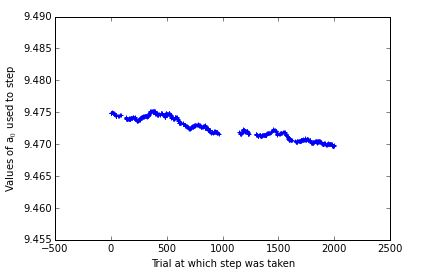Figure 1: Plot of steps taken by the "random walker" representing the parameter $$a_0$$.  The MCMC process is causing the walker to search around the best value for this parameter while looking at the Likelihood at each step.  The gaps represent iterations of the the MCMC process when this specific parameter was not changed.
As you can see in Figure 1, the "walker" moves vertically on the plot over time.  Some values seem to be repeated.  This is the Bootstrapping application of my code.  It is okay for the walker to repeat steps that were chosen previously.  However, because of the MCMC method, the walker gravitates towards values that yield high Likelihoods and probabilities.

From here, I will follow the same analysis I conducted in my Astro-Stats & Python : Bootstrapping, Monte Carlo and a Histogram post.  The histogram of each parameter will reveal the probability distribution function (PDF) of the parameter although the PDF is not explicitly plotted.  Then the cumulative distribution function (CDF) will reveal where the peak of the probability distribution function is as well as the interval for which 68.3% of the central area under the PDF curve is accounted for.  (This is explained thoroughly in my Grad level Intro to Astro-Stats for Undergraduates, cont. post with an example given to apply the concept.)

Despite similar analyses, the results are tremendously different now though.  Because I have now used the LM statistical method first, my MCMC didn't have to waste time in finding the vicinity of the best fitting parameters and subsequently the confidence interval.  This time, my MCMC process devoted 2,000 trials solely towards searching around the best fitting parameters.  This distinction is evident if you compare the uncertainties of the 3 parameters in that post with the 3 parameters' confidence intervals in this post.  This is why I set the "par_sigmas" so low.  Because the "walker" is so close to the best values with the highest Likelihoods, the walker will refuse to make steps that are very far away (where there are much lower Likelihoods).  Therefore, it is best to tell the walker to attempt to make very small steps.  The walker will be more willing to accept very small steps than larger ones and thus the acceptance rate has a chance at being above 40%.

I will now just show the rest of the results.  At a later time, I will run the same code and explain what is happening when the data follows a Gaussian profile, as opposed to my current example's trinomial of second degree profile.  But if you are just too eager to wait and see, you can run my code again with the "sim_data_gauss" option set to "True".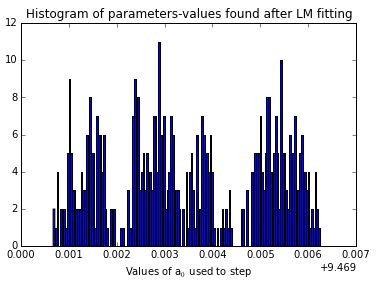Figure 2: The Y-axis is the number of times that that value appeared during the 2,000 trials.  The highest bin(s) seems to be around 0.003 + 9.469, which is 9.472.Figure 3: As the histogram for $$a_0$$ implied, 9.472 was calculated to be the best value for this parameter.  This is good because the true value was 10.  The red error bars represent the confidence interval, i.e. the $$\pm$$ uncertainties.  The green line shows that the CDF goes from 0 to 1 (because it's a type of probability distribution function). It also helps guide your eye towards seeing the X-axis value that is chosen.Figure 4: Plot showing the changes made to parameter $$a_1$$ throughout the Random Walk.Figure 5: The highest bin(s) conspicuously suggest that a number near 0.003 + 499.997 (which is 500) is the best value.Figure 6: Look at those low uncertainties!  Talk about precision, eh!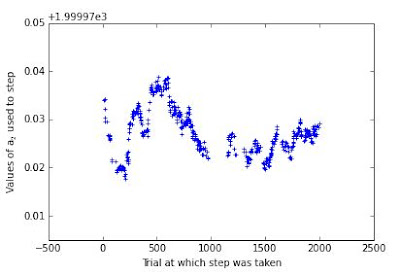Figure 7: Plot showing the changes made to parameter $$a_2$$ throughout the MCMC process.Figure 8: The highest bin(s), i.e. the highest probabilities and frequencies, are around 0.015 + 1999.98, which is 1999.995.Figure 9: I must say, I am proud of this one.  Beautiful plots can make all of that hard work seem worth it.  And oh boy! that precision though!

### Part II : Astro-Stats & Python : Lev-Marq to Markov Chain Monte Carlo and Bootstrapping

Now, that I have reviewed the concepts in the previous posts, I'll get straight to the fun stuff.

The objective of this exercise is essentially the same as my previous posts: determine the best fitting parameters and their confidence intervals as I fit to realistic data.

The methodology behind this exercise is an accumulation of two of my previous exercises:

As always, I invite the reader to follow along on your own computer if you have Python installed.  But things have gotten a little more complex than my last examples.  You'll need to download TWO scripts now in order to witness the full power of my code.
You can copy my scripts from here (LM) and here (MCMC) then paste them into your own two scripts.  Or, you can use the "wget" command, in the Terminal, on these links to immediately download my files.  Make sure that both files are in the same folder!!!!!!

>>>execfile('mcmc_walk.py')
>>>walk_mcmc(x_vals = np.arange(20),   data_sigmas=2,  pars=np.array([10, 500, 2000],dtype=float),   pars_g = np.array([90, 200, 2], dtype = float),   par_sigmas=np.array([.00009, 0.00009, 0.0009], dtype=float),   trials = 2000,   sim_data_gauss = False,   plot=True)

>>># feel free to run this "walk_mcmc" command as many times as you want. Also, feel free to change things if you are familiar with Python.  In order to see ALL of the plots, there is a condition that must be met that I have not mentioned yet.  So, don't worry if you run the "walk_mcmc" function a couple times and have yet to see ALL of the plots.

In this example:
• x-axis values : numbers 0-19
• "data_sigmas" uncertainties of data points, i.e. measurements: 2 (for each data point)
• true parameters : the numbers 10, 500 and 2000
• initial guess at parameters : the numbers 90, 200 and 2
• number of trials for MCMC : 2,000
• "sim_data_gauss" simulated data set used : polynomial + noise  (Refer to 1st bullet point in Astro-Stats & Python : Recap for methodology of simulated data)
• "par_sigmas" uncertainties of the 3 changing parameters in MCMC : 0.0009 . This is extremely small for reasons soon to be explained.

Let's first take a look at how the data looks, which follows the polynomial $$y = a_0 + a_1 x + a_2 x^2$$.Figure 1: Plot of data (dots) and the data without noise (line). Y-axis is Measurement and X-axis is simply the x-values.  Because the uncertainty of each "measurement" is 2, the dots actually do deviate from the line.  That is not very discernible in this plot because I did not zoom in.
When you run the code, the LM statistical method in one of my functions will attempt to find the true parameters.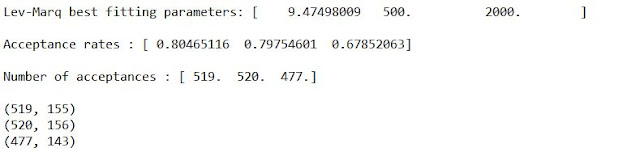Figure 2: My LM fitting function did a great job this time in find the best fitting parameters!
The "acceptance rates" are the ratios of the number times a parameter was changed compared to the number of times the MCMC attempted to change the parameter.  From the "Random Walk" perspective, this is the ratio of the number of times that the person took a step to the number of times the person thought about taking a step.  In this case, there are 3 random walkers because of the 3 parameters.
The criterion for seeing ALL of the plots is that every parameter's acceptance rate must be above 40%.  This is because a good metric for determining if the MCMC did well in ascertaining the confidence interval is if the "walker" accepted 40% of the steps that he/she considered.  There can be a variety of reasons why the MCMC may not do well.  The primary reason for doing badly is the user's choice of "par_sigmas."  If the acceptance rate is < 40% for a parameter, then the user should decrease the sigma for that parameter.  If the rate is ~100%, the sigma for that parameter should increase.  I am currently not sure what should be one's specific threshold for determining what qualifies as being near 100%.Figure 3: Fitting of LM found parameters to original data.  The fit looks great.  Because we actually know the true values of the parameters, it was already apparent that I would have a good fit by the information in Figure 2.

I think that was enough to absorb for one blog post.  I'll continue next time with the rest of the results of this specific execution of my code.

to be continued...

## Friday, July 17, 2015

### Part I : Astro-Stats & Python : Lev-Marq to Markov Chain Monte Carlo and Bootstrapping

Oh, how I am excited to tell you about this script!  I'll briefly reiterate the concepts employed for this exercise and then I'll just jump right into my code and results.

### Levenberg-Marquardt (LM)

LM Statistics is very useful for determining the best fitting parameters.  My function, using this statistical method to fit my data, finds the combination of values for the parameters that yields the least $$\chi^2$$ after trying many different values for each parameter.  This method requires an initial guess for the parameters.  Consequently, if one's hypothesis is far from the truth, the calculations of this LM method may claim that the best parameters are somewhere completely "out of the ballpark".  This means that one should make a somewhat good guess when inputting your priors (or initial parameters). Refer to my previous post Astro-Stats & Python : Levenberg-Marquardt Statistics for further explanation and an exemplary application of this method.

### Markov Chain Monte Carlo (MCMC)

The MCMC method is excellent for finding the confidence interval of each parameter after the best fitting parameters have been found.  From one perspective, this method is also capable of finding the best fitting parameters as well.  However, when the "Random Walk" occurs and my code is checking the Likelihood (for one set of parameters) to see if it should change a certain parameter, it might change that parameter even if the new Likelihood is less than the previous one.  This means that once my MCMC code finds the highest Likelihood--the minimum $$\chi^2$$--and thus finds what is most likely the best parameters, it will continue to "search" around those best parameters to check the gradient of the Likelihoods as the parameters change.  Because it does the search around those values, it may even stumble across another combination of parameter-values that have similar high Likelihoods.  When this happens, it is tough to find which combination of parameters is better than the other.   Essentially, it is great at finding the area around the best fitting parameters but is not superb at pinpointing precisely where the best values are for the parameters.  Refer to my
Astro-Stats & Python : Bootstrapping, Monte Carlo and a Histogram post for a more elaborated description of this methodology and a good example for applying this to data.  (In that example, $$a_0$$ and $$a_1$$ have very low precision--i.e. large uncertainties--associated with what was calculated to be their best values for the fitting.)

### Bootstrapping Statistics

Bootstrapping is any test that utilizes random sampling in which any sampling/outcome can be replaced/repeated.  The randomness component is applied under the Monte Carlo aspect of my work.  The application of this concept of random sampling with replacement is discussed in more detail, once again, in my Astro-Stats & Python : Bootstrapping, Monte Carlo and a Histogram post.  The underlying concept behind this statistical method is that I have created a process that is self-sustaining and thus does not need external input as it produces many outcomes.  This idea of independence and lone-effort alludes to the expression "pulling oneself up by one's own bootstraps".

I think that that was a good way to review on the various things I have been learning before I jump into my next Python script.  Now-a-days, I feel like I am just spitting out new code left and right.  I feel like this course and the Banneker Institute at large is--at a very fast pace--making me substantially more intelligent in Astrophysics research and more productive in Coding.

to be continued...

## Wednesday, July 15, 2015

### Astro-Stats & Python : Levenberg-Marquardt Statistics

Another exciting day in my first course in Grad level Astro-Statistics.  Today I'll discuss a simple exercise that was great in helping me comprehend and apply Levenberg-Marquardt Statistics.

As always, you can follow along and run my code if such is your heart's desire.  However, this time I wrote code in an IPython Notebook.  You can just look at it on my Github page here, or if you have Anaconda installed onto your computer you can play with it yourself if you copy the file from here and paste into your own script.

As usual, I must first simulate data as if I collected them from some star or astrophysical source.  I did this with a random number generator (of a Gaussian distribution) in python which acted as my "noise".  From an astronomer's perspective, noise is just unwanted, additional signal/light from somewhere in space or on Earth that was measured by the telescope's camera.  When observing a star in outer space, you only want your telescope to receive the light from that specific star.  This does not happen because of how the earth's atmosphere alters and adds on to the measurements of that star's light as that light travels from outer space to earth's atmosphere to the Earth's surface.Figure 1: Plot of simulated data.  Just by looking at the plot, you can see why the shape of a plot that follows a Gaussian distribution is commonly referred as a bell curve.
In previous posts, I generated data by using a polynomial equation where the user determined how many coefficients were involved and thus how many terms where in that equation.  Subsequently, a random number would be added to the equation to make it more realistic and unpredictable.  For this exercise I use a different equation that specifically follows a Gaussian distribution.  Furthermore, at the end of this equation I still added noise by using a random number generator.  So now, I have data that abides by a Gaussian along with the confidence interval (or uncertainties) of the measured data points abiding by a Gaussian as well.  My Gaussian equation is
$G(x_i) = A e^{-\frac{1}{2}\Big( \frac{B-x_i}{C} \Big)^2 } + E$
where G is a function of the x-axis values $$x_i$$, A is for the amplitude, B is the centroid of the Gaussian, C is the width and E is for the offset.  and E are known as free parameters and are usually insignificant when analyzing astrophysical data.  This equation will also serve as my model for the Levenberg-Marquardt (LM) component of this exercise.

Now that I have my simulated data and I've chosen my model, we must understand how to perform the least-squares fitting on this data.  In this case, I must find the least $$\chi^2$$ in order to find the best fitting parameters A, B, C and E for my data.  The equation is
$\chi^2 = \sum_{i}^n \Big(\frac{D_i - G_i}{\sigma_{D_i}} \Big)^2$

where $$D_i$$ is the measurement (or data point), $$G_i$$ is the model's result and $$\sigma$$ is the uncertainty of the measurement $$D_i$$.  There is a standard python function that I use to find which combination of values for the 4 parameters will yield a $$G_i$$ that produces the least $$\chi^2$$.  That function is called "leastsq." However, I first must create a function that would input the residual into "leastsq" function:
$R = \frac{D_i - G_i}{\sigma_{D_i}}$
where is the residual.

In order to begin utilizing these equations, I must make initial guesses as to what the value of the parameters are.  This will result in the first $$G_i$$ calculated and thus the first residual and $$\chi^2$$ will be found.  From there, the program will continue to try different values and gravitate towards values that yield a smaller and smaller $$\chi^2$$.

For this example, I guessed that the parameters were A = 20, B = 7, C = 5 and E = 9.   The true values of the parameters were A = 10, B = 5, C = 2 and E = 4.

After finding the best values for my 4 fitting parameters, I plug those values into my model and check to see how well this specific model aligns with the data.Figure 2: Top- my code showing the values that yielded the least $$\chi^2$$, which consequently fits the data better than any other combination of values tried.  Bottom- Plot of original data and the fit for that data.  The fit seems to be incredibly agreeable with the data.

In summary, in order to use the powerful statistical method derived by Levenberg and Marquardt, I had to make initial guesses for my parameters, use the $$\chi^2$$ equation and find the combination of parameter-values that yielded the least $$\chi^2$$.  LM statistics allowed me to find the best fitting parameters for my data.  But it did not provide the confidence interval of those parameters.   Knowing the uncertainty of the evaluated parameters is very important!  This is where Monte Carlo (MC) and Bootstrapping statistics can save the day.  If you ever have a complex and large set of data for which you must find a satisfactory fit to, I recommend that you use LM statistics to find the best fitting parameter first and then use MC and Bootstrapping statistics to find the confidence interval of each parameter.

## Monday, July 13, 2015

### Astro-Stats & Python : Bootstrapping, Monte Carlo and a Histogram

As always, feel free to follow along if you have Python installed on your computer.  You can copy my code from here and paste it to your own script or you can use the "wget" command in the Terminal for that same web page.

For this exercise, the objective is the same as the other exercises I have shared: determine the best fitting parameters no matter how many parameters are given.  This time I have completely changed how I deduce the optimal combination of parameter-values that would yield the minimal $$\chi^2$$, i.e. the highest Likelihood, for my statistics.  Last time, I used nested FOR Loops to determine the Likelihood for every pair of parameter-values that I proposed as guesses (and the sample of guesses for each parameter were near the correct value of the respective parameter).  Now, I utilize no Loops and no initial guesses in my calculations to determine the optimal parameter-values.  This was all thanks to the matrix multiplication mathematics that I used.

For now, I'll refrain from explaining the tedious matrix calculations that went into this work.  But I will mention that computer programmers have a tendency to avoid FOR Loops and find an alternative solution to have their code get the job done.  If you do not know what a FOR Loop is do not fear.  It's definition is not significant to the concept of the Astro-Statistical exercise that I am discussing in this post.  Just know that FOR Loops in the Python coding language typically makes the computer take a longer time to complete tasks than when using matrices or arrays to perform the same job.  However, FOR Loops tend to be a more intuitive approach for writing complex code.

Well... let's get to the fun, shall we?

>>>execfile('bootstrap_blog.py')
>>> histog(A_trials_mat, figs, binning=50, plot=True)
>>># then, if you would like ... REPEAT both commands and you'll get different data, results, and plots

As with the other scripts I have shared, my code receives parameters, finds the corresponding polynomial equation, then adds random noise to that equation in order to simulate realistic data that was measured in nature.  The random number/noise generator in my code follows a normal/Gaussian distribution.  This is the primary reason why I use $$\chi^2$$ minimization in the first place.  If you glance back at my Exercise in Likelihood and $$\chi^2$$, continued... post, take note that the probability function explained there is for finding probabilities within information (in this case, the noise) that is of a normal distribution.  That probability function is how I derived $$\chi^2$$ and Likelihood.

Thanks to matrix multiplication, I found the combination of parameter-values that yield the least $$\chi^2$$.  I perform this action 1,000 times.  However, for each of the 1,000 trials I add different random noise to my initial simulated data and then find another combination of parameter-values.  For this example, 3 parameters were used $$a_0$$, $$a_1$$ and $$a_2$$.  (In this example, the true values of the parameters were 1, 0 and 2 respectively.)  By the end, I will have 1,000 combinations of values for the parameters.

The idea of using random numbers to generate noise (in each trial) exhibits the Monte Carlo aspect of this exercise.  The histogram will illustrate how the Bootstrap concept is applied in this exercise.Figure 1: Histogram illustrating the value of $$a_0$$ that was deduced and the number of trials that that value reappeared.  The x-axis is a sample of 1,000 $$a_0$$ values that were deduced.

According to Figure 1, the value that re-emerges (for yielding the least $$\chi^2$$) the most is a number near 1.20.  Using a histogram to illustrate this result obscures the precise numbers that were evaluated as yielding the least $$\chi^2$$.  Nonetheless, the histogram perspective is a very useful graphic for showing the results because, actually, there were a plethora of distinct $$a_0$$ values found throughout the 1,000 trials.  Thus, the histogram actually gives me an estimate of which numbers were repeated.  For example, Figure 1 says that values of $$a_0$$ between 1.10 and 1.20 were found in ~65 trials.  This idea that a number/outcome is allowed to reappear throughout a consecutive succession of trials alludes to Bootstrapping statistics.  In other words, an element within a sample of elements may be selected more than once.  Furthermore, I am employing the concept of random sampling with replacement under Bootstrapping statistical methods.

From then on, I followed the same methodology I described in my Grad level Intro to Astro-Stats for Undergraduates, cont. post.  The y-axis values are added, so as to obtain the integral of the probability distribution function that is implicitly demonstrated by the histogram's data.

I have yet to show the rest of my results for all 3 parameters.  And I understand that it must have been just eating you up inside to be kept in such suspense while reading the previous two paragraphs.  So, for the satisfaction of your curiosity, I will show the rest of my results.  However, remember that the results change every time the code is ran, so it is highly unlikely that you would end up with the exact same data, plots, and results.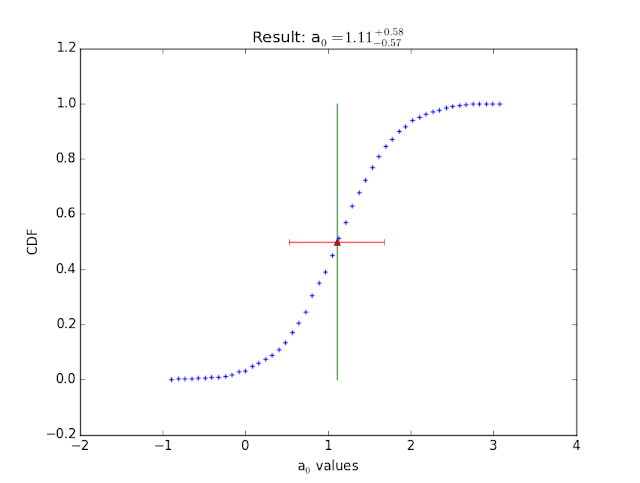Figure 2: The optimal value of $$a_0$$ was determined to be 1.11 with relatively large uncertainties.  Because the true value is 1.0, it would have been nice to have uncertainties near 0.15.  Despite that, I'm content with this.Figure 3: Histogram for resultant $$a_1$$ values evaluated via Monte Carlo and Bootstrapping Statistics.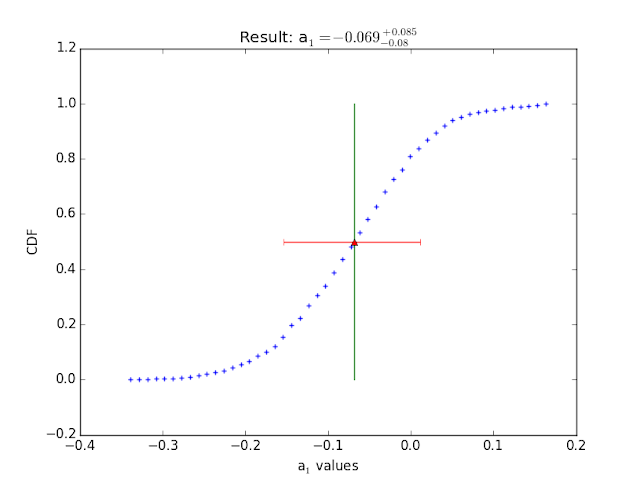Figure 4: The optimal value of $$a_1$$ was determined to be -0.069.  Because the true value is 0.0, I'm excited to see the right red error bar encompass 0.0 (on the x-axis).Figure 5: Histogram for resultant $$a_2$$ values evaluated via Monte Carlo and Bootstrapping Statistics.Figure 6:  Okay!  this is just downright beautiful!  The optimal value of $$a_2$$ was determined to be 1.985.  Because the true value is 2.0, I am pretty darn happy to see those super-small uncertainties!

## Saturday, July 11, 2015

### Astro-Stats & Python : Recap

In my Grad level Intro to Astro-Stats for Undergraduates  post and its sequel, I explained the purpose, meaning and results of one exercise assigned to me within the Banneker Institute.  Recently, we did the same exercise but by using a different method--Monte Carlo Bootstrap.

To quickly recap on the previous posts regarding this Astro-Stats coursework:
• I wrote code that could forge a polynomial equation solely based on the number of coefficients you feed the code.  For example, if I give it the numbers 5, 6 and 100, then my codes knows that the highest degree for this polynomial is 2 and therefore the equation is $$y = 5 + 6x + 100x^2$$.  For another example, I can give it the numbers 0, 250.3, -2.76 and 20 and my code will use the following equation in its calculations: $$y = 0 + 250.3x - 2.76x^2 + 20x^3$$.
• When the various "x" values and coefficients are given, the code will simulate realistic measured data with a random number generator (that follows a normal distribution).  Therefore my equation used to simulate the data would be $$y = 0 + 250.3x - 2.76x^2 + 20x^3 +$$ random_number

• In my previous posts, I used an example where only 2 coefficients were chosen $$a_0$$ and $$a_1$$.  The goal is to approximate the value of those coefficients by using the simulated data (that has noise in it).  In order to do this, I need to make initial guesses as to what those true values are.  I made ~100 guesses for both coefficients.

• I used $$\chi^2$$ statistics on the simulated data and the 100+ coefficient guesses in order to find the Likelihood of every possible combination those 2 sets of guesses.

• With those Likelihoods stored inside of a matrix, I found the Cumulative Distribution Function of the Likelihoods and subsequently used interpolation to find the value for the coefficient  that was at the peak of the Probability Distribution Function.  This was done for both coefficients.

• Lastly, I found the uncertainty of my newly approximated coefficients.

I'll show the Monte Carlo Bootstrap exercise later.

## Friday, July 10, 2015

### Part VII : High Precision Photometry of Transiting Exoplanets as I currently know it

In Part IV, I posed the following questions but forgot to give my brief answer for it:
"So, how does MINERVA (a ground-based observatory) differ from the Kepler Mission?  Furthermore, why am I looking for 'High Precision' measurements from this MINERVA observatory and not the space-based Kepler data?  "

Both telescopes have a purpose to detect exoplanets.  When discerning that Kepler is a space-based telescope and MINERVA is ground-based, your first impression might be that Kepler is better than MINERVA.  However here are a few facts about MINERVA that indicate why it is simply different than Kepler as opposed to being inferior:
• A multi-telescope array allows for simultaneous exposure of primary bright star and its comparison stars (for which satisfactory comparison stars may sometimes not be in the same field of view for 1 telescope alone).
• Despite how related this concept is to my project specifically, this concept is also very related to differential photometry research in general.  Differential photometry is an immensely beneficial and widely used form of research. I think it's cool that an observatory was made to ameliorate this form of research practice.

• A primary purpose of MINERVA is to ALWAYS achieve a measured Doppler Shift precision of 0.8 m/s or less.
• Radial Velocity Curves will have small error bars!
• This is great for confirming exoplanet candidates as true exoplanet detections that have been observed by Kepler.
• This is also great for confirming false-positive exoplanet detections made by Kepler.  A false-positive detection is when the measurements seem to infer that an exoplanet was observed, however after further observations and/or analysis that object was verified to be something else--such as an eclipsing binary star.

• MINERVA was also built for being capable of detecting super-Earths (exoplanets with 2-5R$$_{\oplus}$$)  within their respective habitable zones.  After being detected with radial velocity measurements, these super-Earths can be characterized further with multi-band photometry.  This feat requires a broadband photometric precision < 1 mmag in the optical wavelengths.
• Aside: remember R$$_{\oplus}$$ represents 1 Earth's radius as if it is a unit of measurement, like the meter.  It is a convenient way to compare the size of other objects to an object (the Earth) that we are familiar with. Furthermore, I will elaborate on multi-band photometry and 1 mmag precision at a later time.

• Now that MINERVA can do such a great job at confirming exoplanet candidates initially observed by Kepler, humanity can move quicker in finding planets that may already harbor Life.  Surely, planets that have satisfactory conditions to sustain life will be the first among a list of destinations that astronauts will travel to when long distance space travel becomes more common and feasible.

• Another primary purpose of MINERVA is to detect and confirm exoplanets with small radii.  This is difficult to do because of how small the "dip" of a light curve is for small transiting exoplanets.  In Part IV,  I stated "there are more planets with a radius < 4R$$_{\oplus}$$ than there are larger ones in our Milky Way Galaxy.  This means that a project dedicated to finding exoplanets should certainly be equipped to find planets with a relatively small radius (~Earth size) and low mass."
• This is one reason why the MINERVA project is so focused on achieving high precision measurements.

• It's a ground based telescope.  It costs MUCH less to construct.  It costs MUCH less operate.  Therefore... More astronomers have access to use it for their specific research purposes.  This means students of all ages have a better chance at earning time on this telescope for their research needs as well!
How great is it that I, an undergraduate, can use highly sophisticated technology partially owned by Harvard University to conduct research that will actually benefit the astronomy community at large!  It is because of the access that telescopes like MINERVA provide to the younger astronomy community that makes a young person like me be able to make discoveries that are actually relevant and significant to this well-respected field of highly-educated philosophers of the Universe. Yay! for Opportunity.  And speaking of "access", the MINERVA (Planewave CDK 0.7m) telescopes were made to be wheelchair accessible.  Yay! for social justice and awareness of abilism.  Yay! for the astronomy community respecting the ideas and research of its youngest members.

Sorry for the spontaneous outbursts of celebrations.  I guess I was in a grateful mood.  I think now is as good of a time as any to tweet what the Adler Planetarium deems #science4everyone.

## Tuesday, July 7, 2015

### Methodology &

#### Differential Photometry cont.

A professor at RIT used real data to explain how Differential Photometry is analyzed.  The following plot illustrate the measurements taken after data reduction.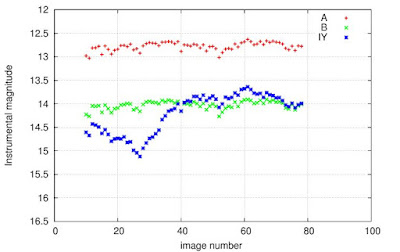Figure 1: Raw photometry of three stars plotted in this light curve.  "Magnitude" is another unit of measurement for a star's brightness.  The Instrumental magnitude is the magnitude, or flux, measured by a camera on Earth.  This measurement of brightness can differ if the Earth was much closer or much farther away from these stars.    Picture Credit: RIT
First of all, be mindful of the fact that light curves usually have "days" or some familiar unit of time for the x-axis.  "Image number" is, in essence, a unit of time but the analysis would be much more thorough and more information would be gathered if a familiar unit of time was utilized.  With this choice of axis, a variety of questions are left unanswered because of the author's reluctance to simply show the time stamp of each image number.  How do we know that image 20 was taken the same night as image 40?  Does the flux of star IY vary significantly over the course of days, weeks or hours?  Are the time intervals between when each image was taken the same?  (The author actually does show a more descriptive choice of x-axis later at the bottom of his/her web page.)

Based on the plot, it seems like all three of the stars are variable, i.e. for some reason they change in brightness over time.  The next plot will show how such a conjecture would not be true.Figure 2: Light curve of photometric measurements after utilizing the two comparison stars A and B.  As described in the legend of the plot, all instrumental magnitudes were subtracted by the corresponding instrumental magnitude of star A at that time.  This is why all of the values for star A are at magnitude 0.  Star A is being used the comparison star.  Star B is another comparison star used to confirm the assumption that both stars A and B are not variable stars.  Picture Credit: RIT
As stated in my previous post, comparison stars are useful for determining when the Earth's atmosphere is interfering with the incoming photons from stars in such a way that makes the star look like it is changing in brightness.  Therefore, it is wrong to trust the raw data in Figure 1 and conclude that all three stars vary in brightness over time.

In Figure 2, the flux of star B remains nearly constant--relative to star A.  This means that the initial measured flux of stars A and B varied in the same amount at the same times.  Because it is highly improbable that both stars would fluctuate at exactly the same times and with the same amplitude of fluctuation, it is safe to assume that the variability seen in Figure 1 is due to the Earth's atmosphere.  There are a few bumps in the Figure 2 light curve for star B.  However, those bumps are so small that they are insignificant when searching for a variable star.  The flux of star B is certainly consistent enough for this example.  However, if one was skeptical and wanted to more evidence that both stars A and B are good comparison stars (i.e. remain constant in flux during the observation), then more comparison stars should be observed simultaneously with the primary star of interest.  Once more non-variable stars are observed and their flux plotted, one would see how the data points for each comparison star remains sufficiently constant throughout the observation.